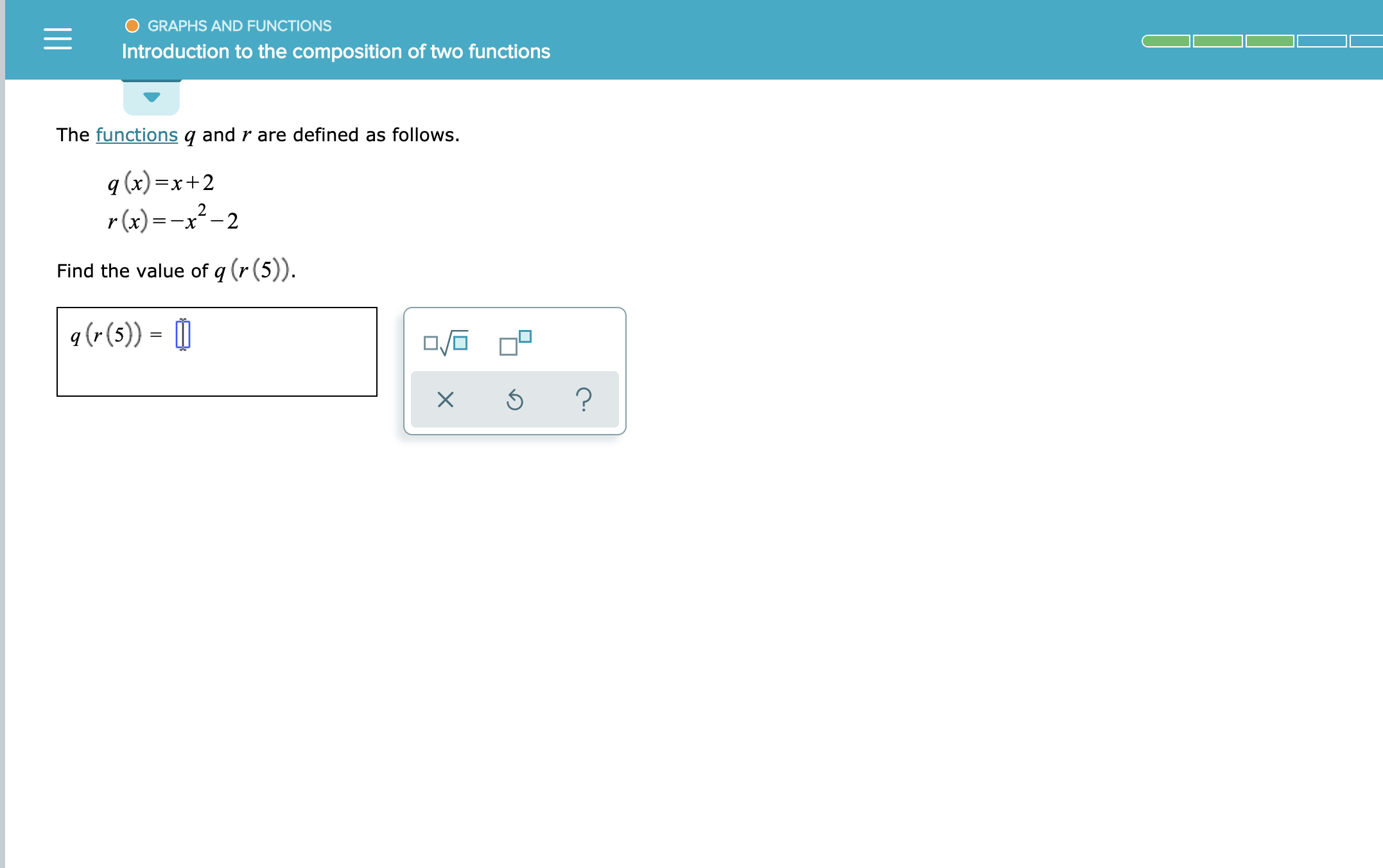# GRAPHS AND FUNCTIONSIntroduction to the composition of two functionsThe functions q and r are defined as follows.q (x)x+22=-Xr(x)-x-2Find the value of q (r(5)).q (r(5))?X

Question
3 views

SEE ATTACHMENThelp_outlineImage TranscriptioncloseGRAPHS AND FUNCTIONS Introduction to the composition of two functions The functions q and r are defined as follows. q (x)x+2 2 =-X r(x)-x-2 Find the value of q (r(5)). q (r(5)) ? X fullscreen
check_circle

Step 1

The given functions, q and r are,

Step 2

Substitute the value 5 for x in the function r an...

### Want to see the full answer?

See Solution

#### Want to see this answer and more?

Solutions are written by subject experts who are available 24/7. Questions are typically answered within 1 hour.*

See Solution
*Response times may vary by subject and question.
Tagged in

### Functions